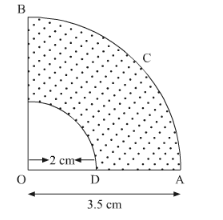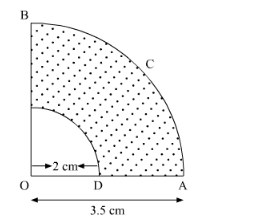# In the following figure, OACB is a quadrant of a circle

Question:

In the following figure, OACB is a quadrant of a circle with centre O and radius 3.5 cm. If OD = 2 cm, find the area of theSolution:

It is given that OACB is a quadrant of circle with centre at O and radius 3.5 cm.(i) We know that the area of quadrant of circle of radius r is,

$A=\frac{1}{4} \pi r^{2}$

Substituting the value of radius,

$A=\frac{1}{4} \times \frac{22}{7} \times 3.5 \times 3.5$

$=9.625 \mathrm{~cm}^{2}$

Hence, the area of $\mathrm{OACB}$ is $9.625 \mathrm{~cm}^{2}$.

(ii) It is given that radius of quadrant of small circle is 2 cm.

Let the area of quadrant of small circle be $A^{\prime}$.

$A^{\prime}=\frac{1}{4} \pi r^{2}$

$=\frac{1}{4} \times \frac{22}{7} \times 2 \times 2$

$=3.14 \mathrm{~cm}^{2}$

It is clear from the above figure that area of shaded region is the difference of larger quadrant and the smaller one. Hence,

Area of shaded region $=A-A^{\prime}$

$=9.625-3.14$

$=6.485 \mathrm{~cm}^{2}$